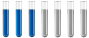## The ionization constant of water and its ionic product

Chemistry and homework help forum.

Organic Chemistry, Analytical Chemistry, Biochemistry, Physical Chemistry, Computational Chemistry, Theoretical Chemistry, High School Chemistry, Colledge Chemistry and University Chemistry Forum.

Share your chemistry ideas, discuss chemical problems, ask for help with scientific chemistry questions, inspire others by your chemistry vision!

Please feel free to start a scientific chemistry discussion here!

Discuss chemistry homework problems with experts!

Ask for help with chemical questions and help others with your chemistry knowledge!

Moderators: expert, ChenBeier, Xen

Dhamnekar Winod
Full MemberPosts: 16
Joined: Sat Nov 21, 2020 10:14 am
Location: Mumbai[Bombay],Maharashtra State,India

### The ionization constant of water and its ionic product

The following equilibrium exists.$$H_2O(l)_{\text{acid}} +H_2O(l)_{\text{base}}\rightleftharpoons H_3O^+(aq)_{\text{Conjugate acid}} + OH^-(aq)_{\text{Conjugate base}}$$
The equilibrium constant$$(K)=\frac{[H_3O^+][OH^-]}{[H_2O]}=[H^+][OH^-]=K_w=?$$

Now, how is $$(K_w)= {[H_3O^+][OH^-]}$$= (1.00e-7)² M =1.00e-14 M²
Any science consists of the following process. 1) See 2)Hear 3)Smell,if needed 4)Taste, if needed 5)Think 6)Understand 7)Inference 8)Take decision [Believe or disbelieve, useful or useless, true or false, cause or effect, any other criteria]
ChenBeier
Distinguished MemberPosts: 493
Joined: Wed Sep 27, 2017 7:25 am
Location: Berlin, Germany

### Re: The ionization constant of water and its ionic product

Its because the dissociation is like this. In neutral water 10^-7 H+ and also the same amount of OH- in solution.